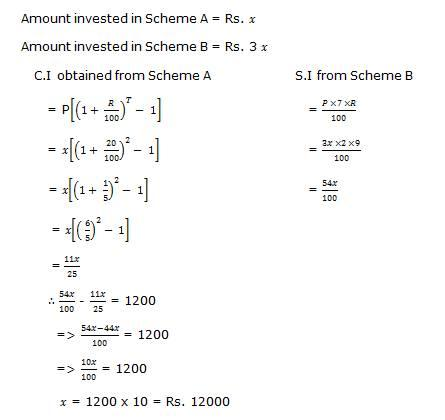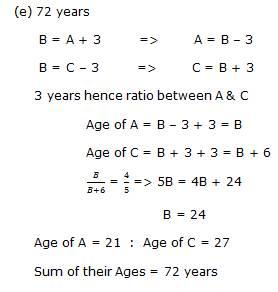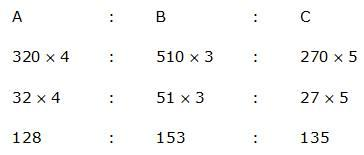## Ratio and Proportion For SBI PO : Set – 09

1) The ratio of the monthly incomes of Sneha, Tina and Akruti is 95:110:116. If Snehas annual income is 3,42,000, what is Akrutis annual income?

a) 3,96,900

b) 5,63,500

c) 4,17,600

d) 3,88,000

e) None of these

c)

Sneha’s annual income = Rs.3,42,000

Sneha’s monthly income = 3,42,000/12=28,500

A monthly income = (28500/95)*116

A annual income = 34,800*12=Rs.4,17,600

2) The ratio of the number of students studying in Schools A, B and C is 4 : 8 : 3 respectively. If the number of students studying in each of the schools is increased by 80%, 20% and 60% respectively, what will be the new ratio of the number of students in Schools A, B and C.

a) 8 : 3 : 4

b) 4 : 2 : 3

c) 2 : 3 : 4

d) Cant say

e)  None of these

e)

Initial ratio A : B : C  = 4: 8: 3

After Increase -> A : B : C

-> (4+3.2):(8+1.6)+(3+1.8)

-> 7.2 : 9.6 : 4.8

-> 3:4:2

3) Two numbers are in the ratio of 3:5 .If 9 be subtracted from each. Then they are in the ratio of 12 : 23. Find the numbers.

a) 15:28

b) 36: 115

c) 33 :55

d) 60 :69

e) None of these

c)

4) In a class of 75 students, 1/5 th of the total number of girls and 3/5 th of the total number of boys join a cricket club. If the total number of boys joining the club is 27, what is the respectively ratio of the total number of boys total number of girl joining the club?

a) 2:9

b) 3:2

c) 9:2

d) Cannot be determined

e) None of these

c)

5) A mixture contains A and B in the ratio 2 : 5. 7 litres of mixture is replaced by 18 litres of A and the new ratio becomes 8 : 11 respectively. What is the amount of A present in the original mixture?

a) 20 litres

b) 18 litres

c) 44 litres

d) 24 litres

e) None of these

d)

6) The respective ratio of the sums invested for 2 years each, in Scheme A offering20% per annum compound interest (compounded annually)and in Scheme Boffering 9% p.a. simple interest is 1 : 3. Difference between the interests earned from both the schemes is Rs. 1200. How much was invested in Scheme A ?

a) Rs. 10500

b) Rs. 15000

c) Rs. 12000

d) Rs. 12500

e) Rs. 10000

c)7) B is 3 years older than A and B is also 3 years younger than C. 3 years hence, the respective ratio between the ages of A and C will be 4 : 5. What is the sum of the present ages of A, B and C ?

a) 48 years

b) 56 years

c) 63 years

d) 84 years

e) 72 years

e)8) A, B and C enter into a partnership. A contributes 320 for 4 months, B contributes Rs. 510 for 3 months, and C contributes Rs. 270 for 5 months. If the total profit is Rs. 208, find the profit share of the partner A.

a) Rs. 76.50

b) Rs. 64

c) Rs. 67.50

d) Rs. 46

e) None of these

b)A share = (128/416)*208

A share = 64

9) The price of a diamond is proportional to the square of its weight. The diamond accidentally fell and broke into four pieces whose weights are in the ratio of 1:2:3:4. If the price fetched is Rs. 70,000 less than the original price, find the original price?

a) Rs. 100,000

b) Rs. 70,000

c) Rs. 160,000

d) Rs. 10800

e) Rs. 150,000

a)

If we assume weight of diamond 10x, will I come up with 10x because the ratio, given 1: 2: 3: 4 add upto 10.

So, individual weights of diamonds will be x, 2x, 3x and 4x

Original price = K (10x)2

Price for pieces = K (x2 + 4x2 + 9x2 + 16x2)

= K 30x2

Thus change in price us see in 100 Kx2 30Kx2 = 70Kx2 which is given is 70,000

So,the original price is Rs. 100,000

10) RS.73,689/- are divided between A and B in the ratio 4:7. What is the difference between thrice the share of A and twice the share of B?

a) Rs. 35699/-

b) Rs. 46,893/-

c) Rs. 20,097/-

d) Rs. 26796/-

e) Rs. 13,398/-